## Topic: Algebra

Free Printable Worksheets on Algebra

## Algebra: 2 Step Addition & Subtraction Equations (Set 1)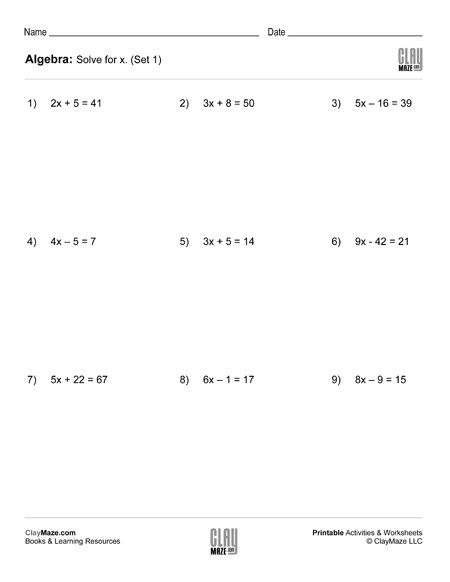This is a worksheet for students learning how to solve equations. Each problem has a combination of multiplication and either addition or subtraction, but division will also need to be used to...

## Algebra: 2 Step Addition & Subtraction Equations (Set 2)Download this worksheet for practice in solving equations. The student is asked to find the value of x in each of the problems....

## Algebra: 2 Step Addition & Subtraction Equations (Set 3)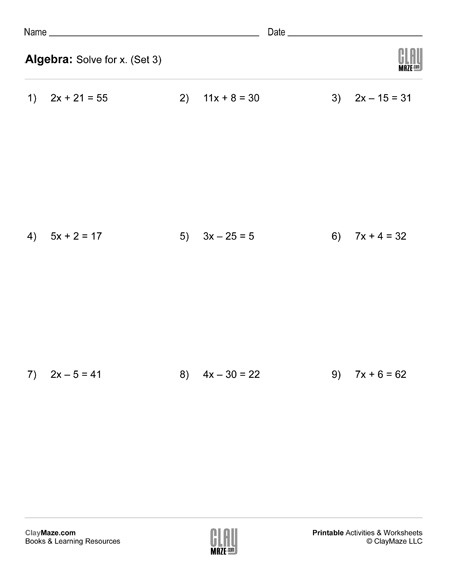Print this practice worksheet for students learning to solve equations. Each problem has a combination of multiplication and either addition or subtraction. Division will also be used to find the...

## Algebra: 2 Step Addition & Subtraction Equations (Set 4)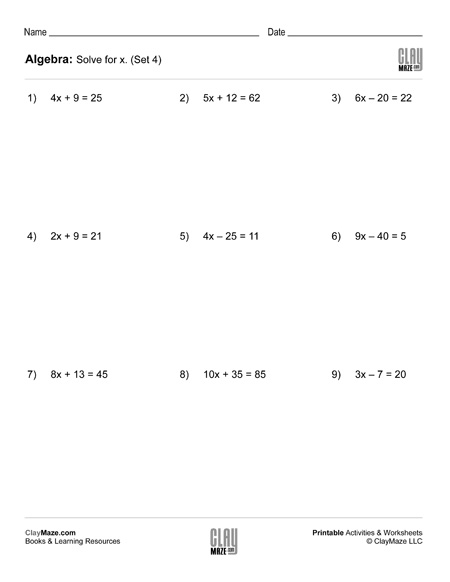Use this worksheet for practice with solving equations. Solve for x in each problem. – 15 problems...

## Algebra: Expand & Combine Like Terms (Set 1)Use the worksheet for practice multiplying expressions and combining like terms....

## Algebra: Expand & Combine Like Terms (Set 2)Algebra worksheet on multiplying expressions in parentheses and combining like terms....

## Algebra: Expand & Combine Like Terms (Set 3)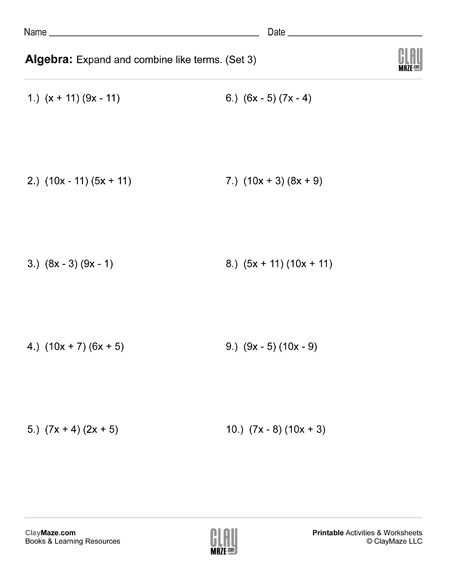## Algebra: Expand & Combine Like Terms (Set 4)Algebra Worksheet – Expand and combine the like terms....

## Algebra: Distribute & Combine Like Terms (Set 1)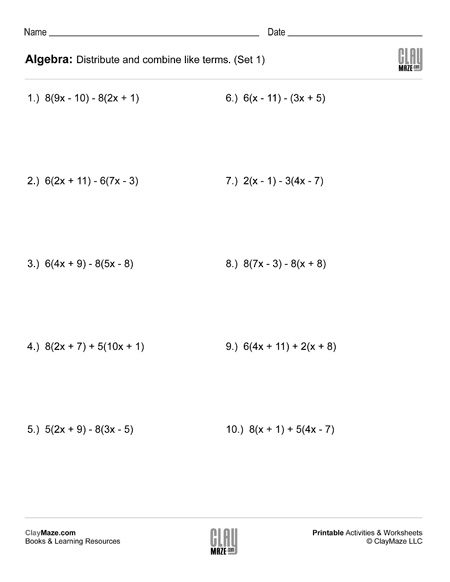Use the worksheet for practice in distributing and combining like terms....

## Algebra: Distribute & Combine Like Terms (Set 2)Algebra worksheet on distributing and combining like terms....

## Algebra: Distribute & Combine Like Terms (Set 3)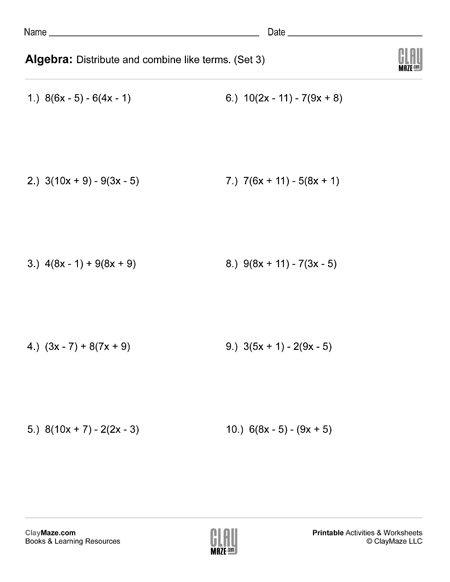Download and print this algebra worksheet on distributing and combining like terms....

## Algebra: Distribute & Combine Like Terms (Set 4)Algebra Worksheet – Distribute and combine the like terms....

## Algebra: 1 Step Addition & Subtraction Equations (Set 1)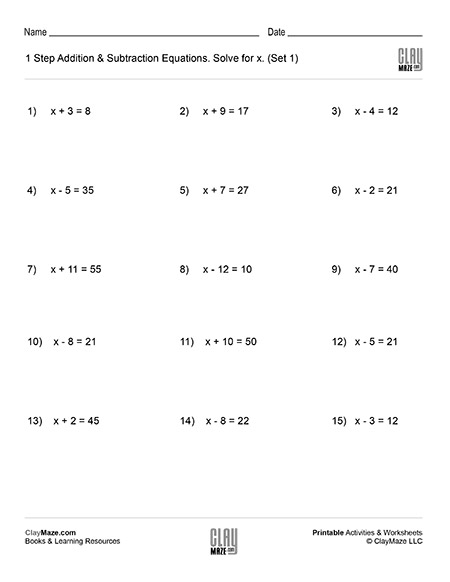This worksheet contains problems that act as an introduction to algebra. The problems are 1 step addition and subtraction equations that ask the student to solve for x. – solutions included...

## Algebra: 1 Step Addition & Subtraction Equations (Set 2)Algebra introduction worksheet. 1 step addition and subtraction equations. – 15 problems with solutions...

## Algebra: 1 Step Addition & Subtraction Equations (Set 3)Intro algebra worksheet: Find the value of x for the problems listed. – 1 step addition and subtraction equations. – with solutions...